# 前言

Python 是一种面向对象、解释型、弱类型的脚本语言，它也是一种功能强大而完善的通用型语言。

Python 的两大特色是清晰的语法和可扩展性：

• Python 的语法非常清晰，它甚至不是一种格式自由的语言。例如，它要求 if 语句的下一行必须向右缩进，否则不能通过编译。
• Python的可扩展性体现为它的模块，Python 具有脚本语言中最丰富和强大的类库（这些类库被形象地称为“batteries included，内置电池”），这些类库覆盖了文件 I/O、GUI、网络编程、数据库访问、文本操作等绝大部分应用场景。

Python 作为一门解释型的语言，它天生具有跨平台的特征，只要为平台提供了相应的 Python 解释器，Python 就可以在该平台上运行。（解释型语言几乎天然是跨平台的。）

## 1.Python Hello World 实例

``````# -*- coding: UTF-8 -*-
# Filename : helloworld.py
# author by : www.runoob.com
# 该实例输出 Hello World! print('Hello World!')
``````

Hello World！

## 2.Python 数字求和

``````# -*- coding: UTF-8 -*-
# Filename : test.py
# author by : www.runoob.com
# 用户输入数字 num1 = input('输入第一个数字：')
num2 = input('输入第二个数字：')
# 求和 sum = float(num1) + float(num2)
# 显示计算结果 print('数字 {0} 和 {1} 相加结果为：{2}'.format(num1, num2, sum))
``````

## 3.Python 平方根

``````# -*- coding: UTF-8 -*-
# Filename : test.py
# author by : www.runoob.com
num = float(input('请输入一个数字：')) num_sqrt = num ** 0.5
print(' %0.3f 的平方根为 %0.3f'%(num ,num_sqrt))
``````

\$ python test.py

4.000 的平方根为 2.000

## 4.Python 二次方程

``````实例(Python 3.0+)
# Filename : test.py
# author by : www.runoob.com
# 二次方程式 ax**2 + bx + c = 0
# a、b、c 用户提供，为实数，a ≠ 0
# 导入 cmath(复杂数学运算) 模块
import cmath
a = float(input('输入 a: '))
b = float(input('输入 b: '))
c = float(input('输入 c: '))

# 计算
d = (b**2) - (4*a*c)
# 两种求解方式
sol1 = (-b-cmath.sqrt(d))/(2*a)
sol2 = (-b+cmath.sqrt(d))/(2*a)
print('结果为 {0} 和 {1}'.format(sol1,sol2))
``````

\$ python test.py

## 5.Python 计算三角形的面积

``````# -*- coding: UTF-8 -*-
# Filename : test.py
# author by : www.runoob.com
a = float(input('输入三角形第一边长: '))
b = float(input('输入三角形第二边长: '))
c = float(input('输入三角形第三边长: '))
# 计算半周长
s = (a + b + c) / 2
# 计算面积
area = (s*(s-a)*(s-b)*(s-c)) ** 0.5
print('三角形面积为 %0.2f' %area)
``````

\$ python test.py

## 6.Python 计算圆的面积

``````# 定义一个方法来计算圆的面积
def findArea(r):
PI = 3.142
return PI * (r*r)
# 调用方法
print("圆的面积为 %.6f" % findArea(5))
``````

## 7.Python 随机数生成

``````# -*- coding: UTF-8 -*-
# Filename : test.py
# author by : www.runoob.com
# 生成 0 ~ 9 之间的随机数
# 导入 random(随机数) 模块
import random
print(random.randint(0,9))
``````

4

## 8.Python 摄氏温度转华氏温度

``````# -*- coding: UTF-8 -*-
# Filename : test.py
# author by : www.runoob.com
# 用户输入摄氏温度
# 接收用户输入
celsius = float(input('输入摄氏温度: '))
# 计算华氏温度
fahrenheit = (celsius * 1.8) + 32
print('%0.1f 摄氏温度转为华氏温度为 %0.1f ' %(celsius,fahrenheit))
``````

celsius = (fahrenheit - 32) / 1.8

## 9.Python 交换变量

``````# -*- coding: UTF-8 -*-
# Filename : test.py
# author by : www.runoob.com
# 用户输入
x = input('输入 x 值: ')
y = input('输入 y 值: ')
# 创建临时变量，并交换
temp = x
x = y
y = temp
print('交换后 x 的值为: {}'.format(x))
print('交换后 y 的值为: {}'.format(y))
``````

x,y = y,x

``````实例
# -*- coding: UTF-8 -*-
# Filename : test.py
# author by : www.runoob.com
# 用户输入
x = input('输入 x 值: ')
y = input('输入 y 值: ')
# 不使用临时变量
x,y = y,x
print('交换后 x 的值为: {}'.format(x))
print('交换后 y 的值为: {}'.format(y))
``````

## 10.Python if 语句

``````# Filename : test.py
# author by : www.runoob.com
# 用户输入数字
num = float(input("输入一个数字: "))
if num > 0:
print("正数")
elif num == 0:
print("零")
else:
print("负数")
``````

``````# Filename ：test.py
# author by : www.runoob.com
# 内嵌 if 语句
num = float(input("输入一个数字: "))
if num >= 0:
if num == 0:
print("零")
else:
print("正数")
else:
print("负数")
``````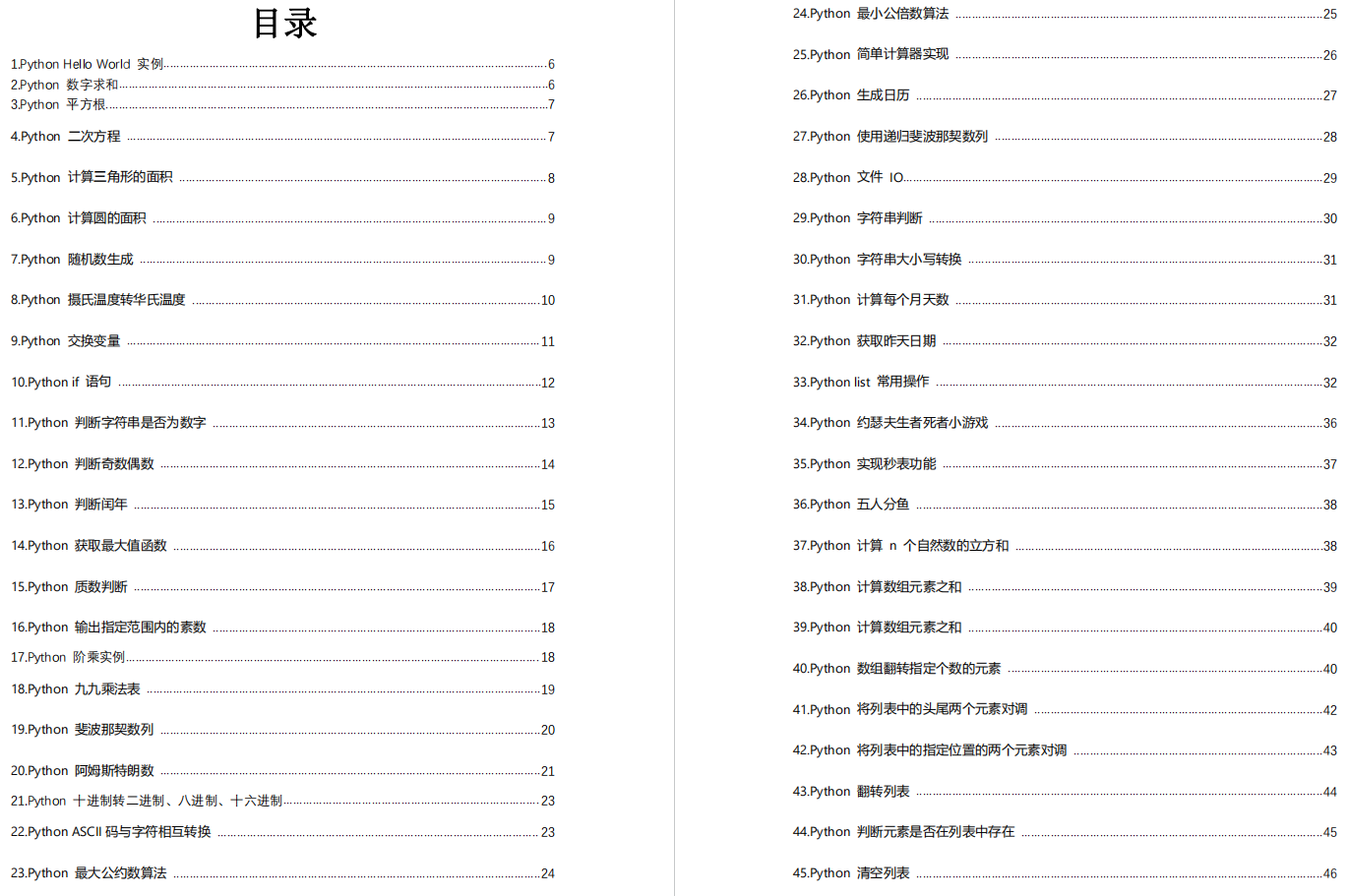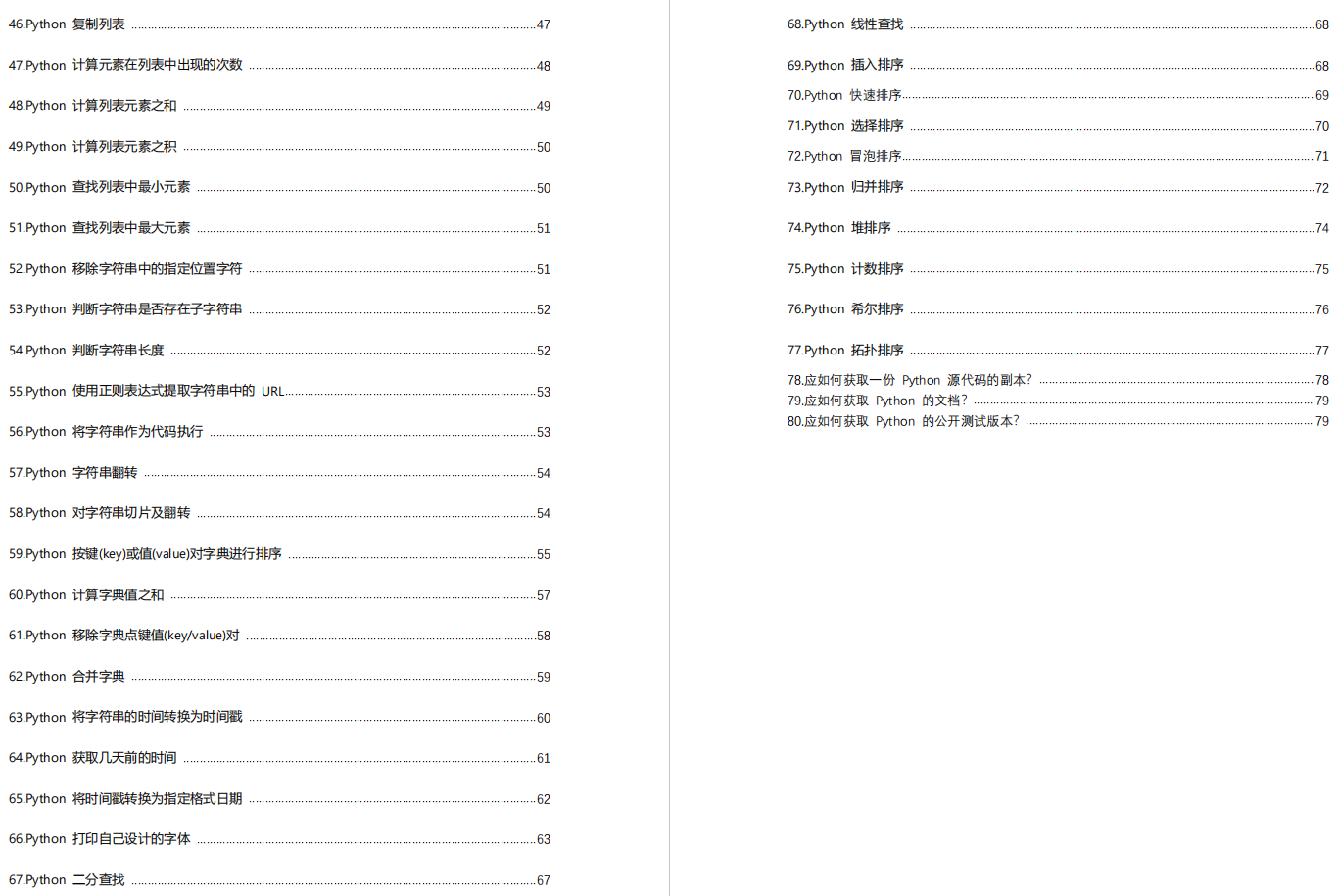# 零基础Python学习资料介绍## 👉Python学习路线汇总👈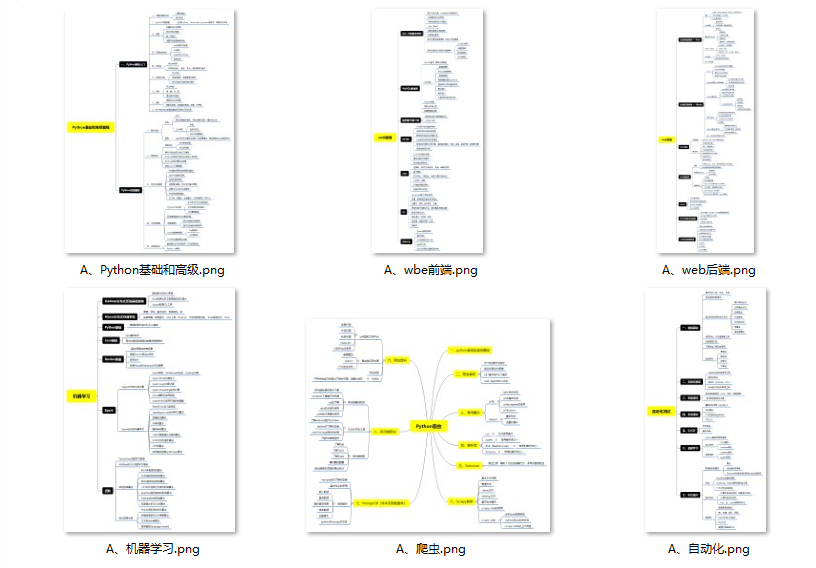## 👉Python必备开发工具👈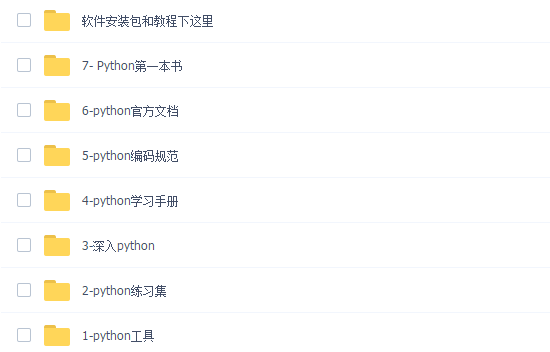## 👉Python学习视频600合集👈## 👉实战案例👈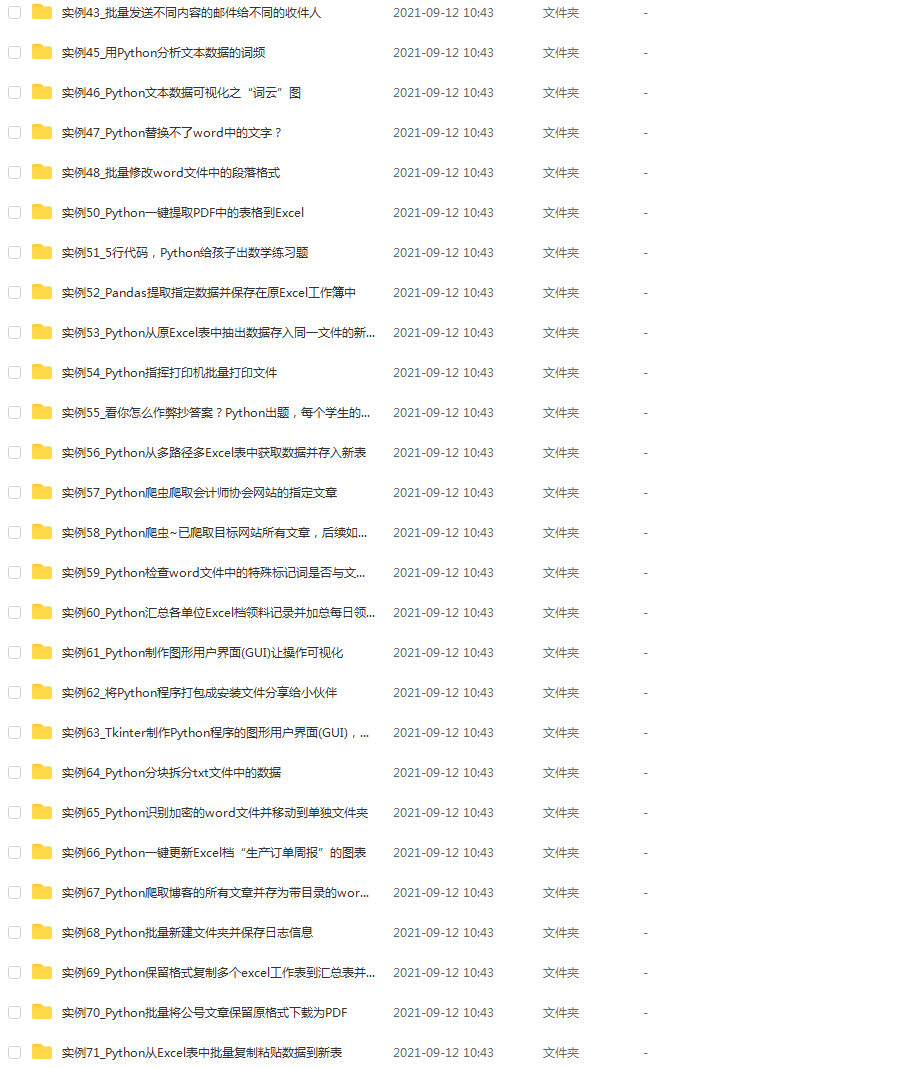## 👉面试刷题👈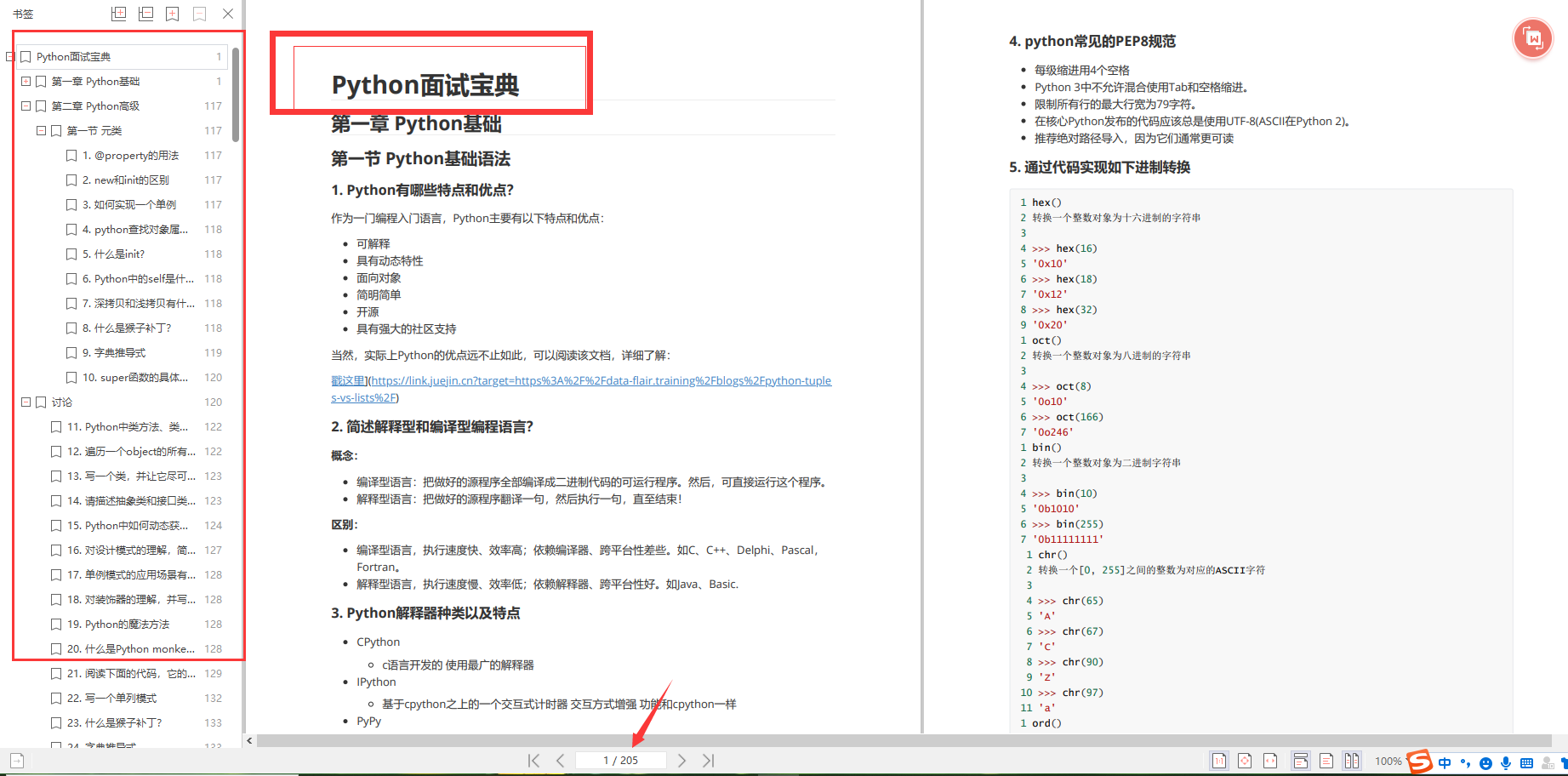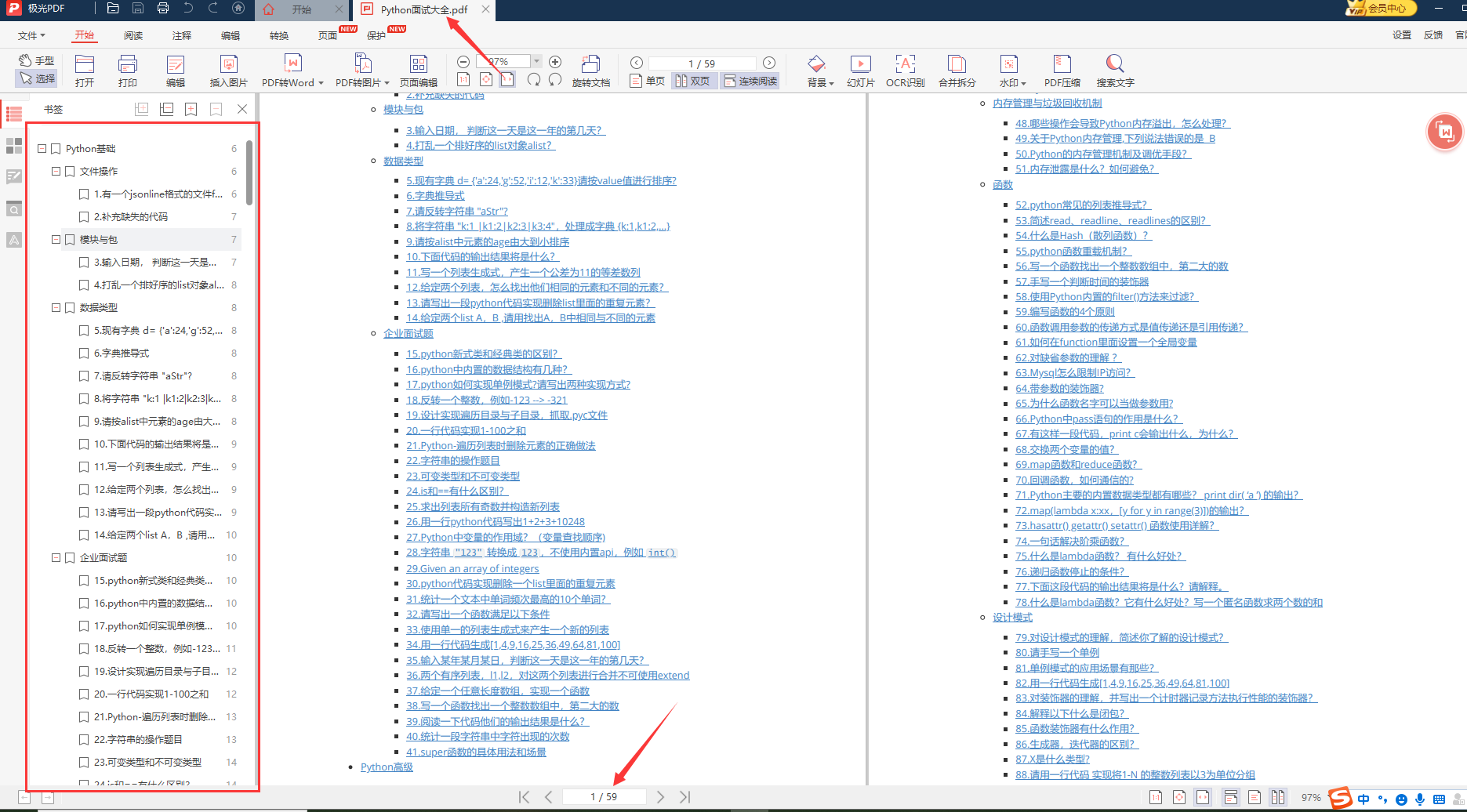# 👉资料领取👈